# 部分和

### 例子：

（数）列是顺序排列的物件（通常是数)。（注意：无穷的项的和是无穷级数

## Sigma

Σ   这符号（英语叫 Sigma）的意思是"加起来"

Σ
n
所以我们把 n 加在一起

### 但n 的值是多少？

4
Σ
n=1
n
这说：n 是从 1 到 4，

### 好了，我们来算……

4
Σ
n=1
n = 1 + 2 + 3 + 4 = 10## 更强大

Σ 能做更强大的事！

4
Σ
n=1
n2 = 12 + 22 + 32 + 42 = 30

4
Σ
n=1
(2n+1) = 3 + 5 + 7 + 9 = 24

3
Σ
i=1
i(i+1) = 1×2 + 2×3 + 3×4 = 20

5
Σ
i=3
i i + 1 = 3 4 + 4 5 + 5 6

## 特性

### 乘以常数特性

ak 可以是 k2k(k-7)+2 或……什么都可以

c 是个常数（像 2-9.1 等），则：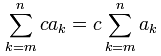### 例子：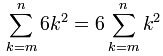### 加或减特性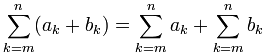### 例子：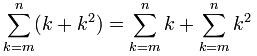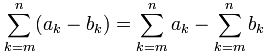## 有用的捷径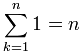把 1 加起来，结果是 n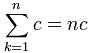把常数 c 加起来，结果是 c 乘 n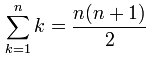把 k 加起来的的捷径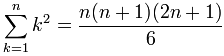把 k2 加起来的的捷径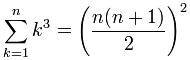把 k3 加起来的捷径

### 例子：你卖园艺砖头。12 + 22 + 32 + ... + 142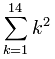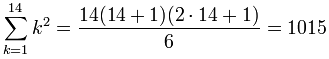### 例子：顾客要讲价。

• 外面的砖头 ￥7 一块
• 里面的 ￥11 一块。• 外面的砖头 = 4×（层的一边的大小 - 1）
• 里面的砖头 = （层的一边的大小 - 2）2

• 价钱（外面的砖头） = ￥7 × 4（层的一边的大小 - 1)
• 价钱（里面的砖头） = ￥11 × （层的一边的大小 - 2）2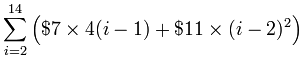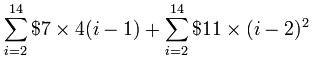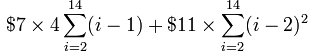• j = i-1
• k = i-2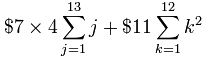（我不算 k=0，因为 02=0）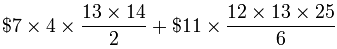\$7 × 364 + \$11 × 650 = \$9,698.00

364 + 650 + 1 = 1015